Forming Differential equations

Chapter 9 Class 12 Differential Equations
Serial order wise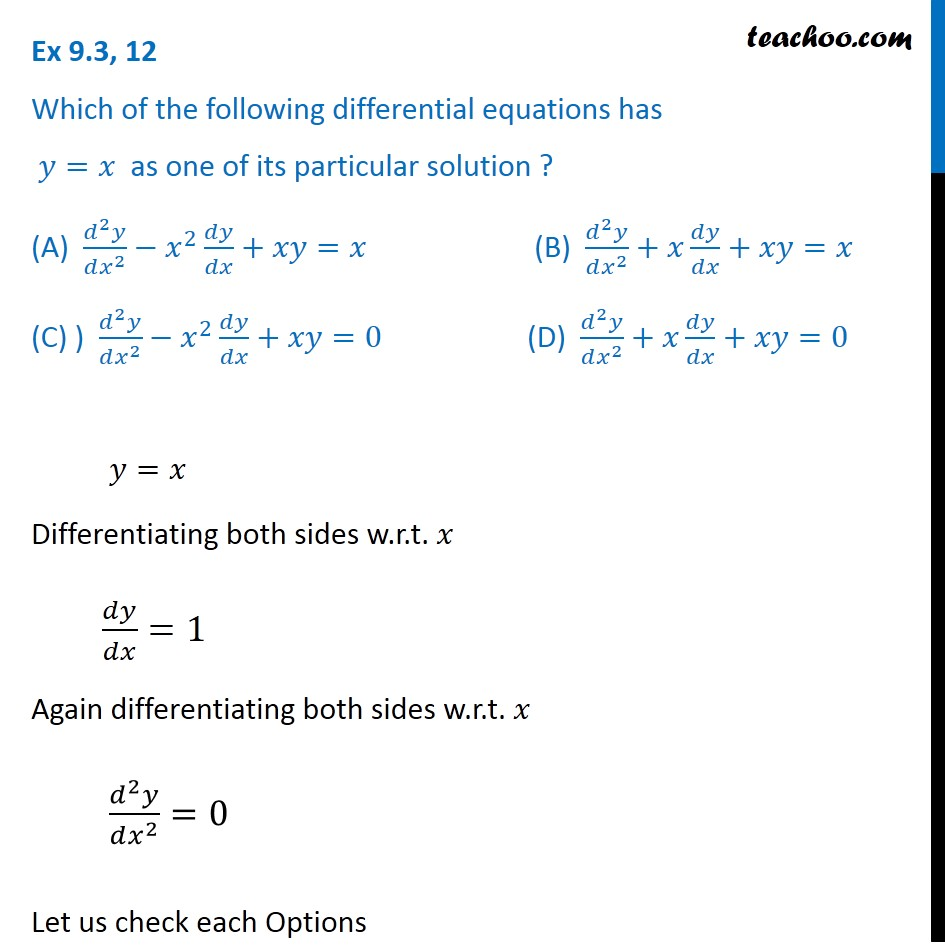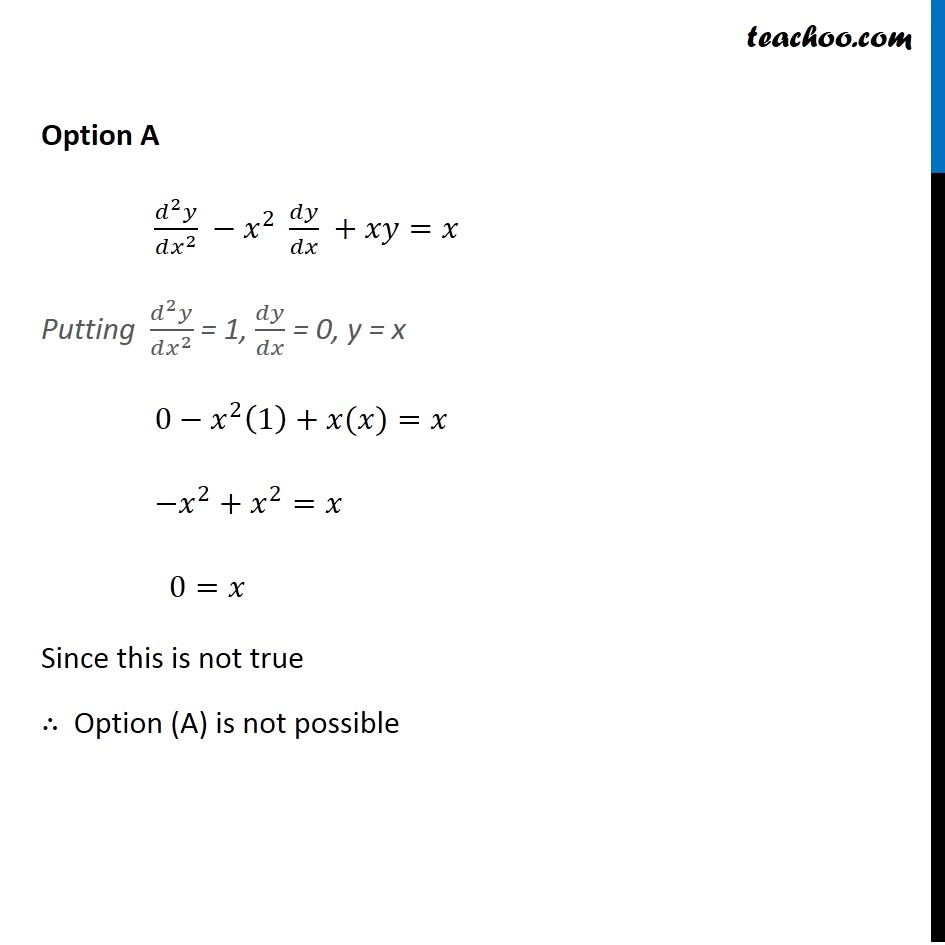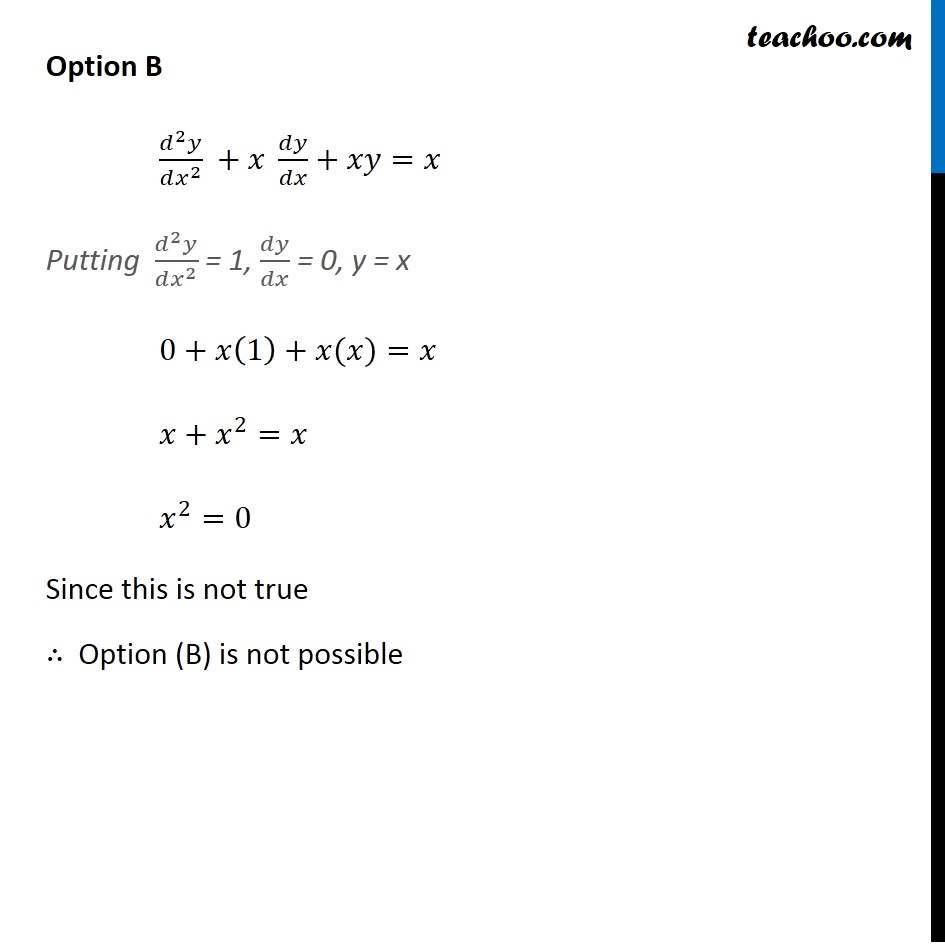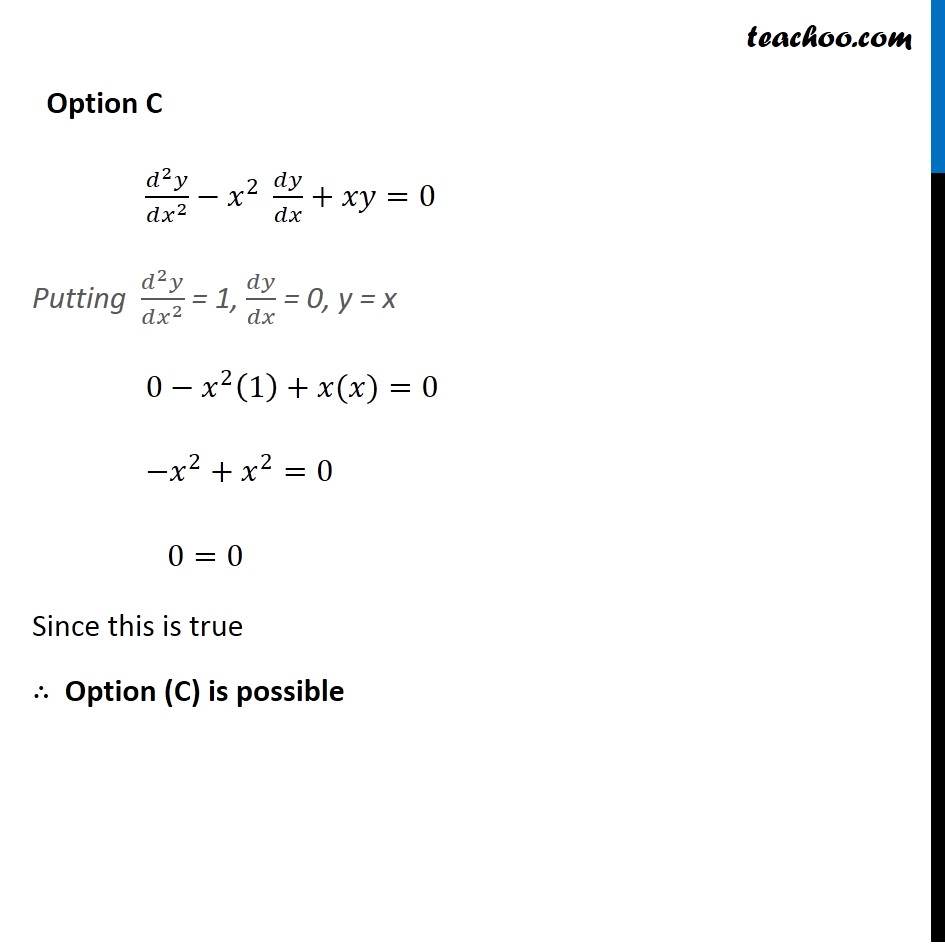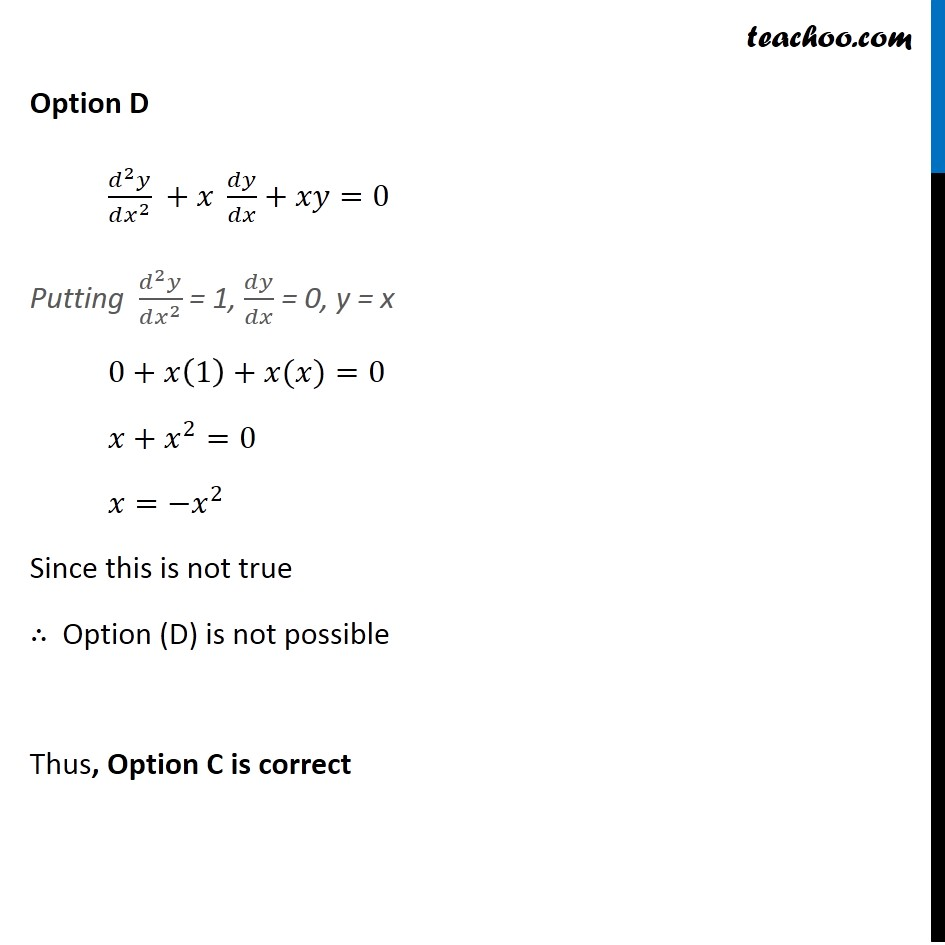Learn in your speed, with individual attention - Teachoo Maths 1-on-1 Class

### Transcript

Question 12 Which of the following differential equations has 𝑦=𝑥 as one of its particular solution ? (A) (𝑑^2 𝑦)/(𝑑𝑥^2 )−𝑥^2 𝑑𝑦/𝑑𝑥+𝑥𝑦=𝑥 (B) (𝑑^2 𝑦)/(𝑑𝑥^2 )+𝑥 𝑑𝑦/𝑑𝑥+𝑥𝑦=𝑥 (C) ) (𝑑^2 𝑦)/(𝑑𝑥^2 )−𝑥^2 𝑑𝑦/𝑑𝑥+𝑥𝑦=0 (D) (𝑑^2 𝑦)/(𝑑𝑥^2 )+𝑥 𝑑𝑦/𝑑𝑥+𝑥𝑦=0 𝑦=𝑥 Differentiating both sides w.r.t. 𝑥 𝑑𝑦/𝑑𝑥=1 Again differentiating both sides w.r.t. 𝑥 (𝑑^2 𝑦)/(𝑑𝑥^2 )=0 Let us check each Options Option A (𝑑^2 𝑦)/(𝑑𝑥^2 ) −𝑥^2 𝑑𝑦/𝑑𝑥 +𝑥𝑦=𝑥 Putting (𝑑^2 𝑦)/(𝑑𝑥^2 ) = 1, 𝑑𝑦/𝑑𝑥 = 0, y = x 0−𝑥^2 (1)+𝑥(𝑥)=𝑥 −𝑥^2+𝑥^2=𝑥 0=𝑥 Since this is not true ∴ Option (A) is not possible Option B (𝑑^2 𝑦)/(𝑑𝑥^2 ) +𝑥 𝑑𝑦/𝑑𝑥+𝑥𝑦=𝑥 Putting (𝑑^2 𝑦)/(𝑑𝑥^2 ) = 1, 𝑑𝑦/𝑑𝑥 = 0, y = x 0+𝑥(1)+𝑥(𝑥)=𝑥 𝑥+𝑥^2=𝑥 𝑥^2=0 Since this is not true ∴ Option (B) is not possible Option C (𝑑^2 𝑦)/(𝑑𝑥^2 )−𝑥^2 𝑑𝑦/𝑑𝑥+𝑥𝑦=0 Putting (𝑑^2 𝑦)/(𝑑𝑥^2 ) = 1, 𝑑𝑦/𝑑𝑥 = 0, y = x 0−𝑥^2 (1)+𝑥(𝑥)=0 −𝑥^2+𝑥^2=0 0=0 Since this is true ∴ Option (C) is possible Option D (𝑑^2 𝑦)/(𝑑𝑥^2 ) +𝑥 𝑑𝑦/𝑑𝑥+𝑥𝑦=0 Putting (𝑑^2 𝑦)/(𝑑𝑥^2 ) = 1, 𝑑𝑦/𝑑𝑥 = 0, y = x 0+𝑥(1)+𝑥(𝑥)=0 𝑥+𝑥^2=0 𝑥=−𝑥^2 Since this is not true ∴ Option (D) is not possible Thus, Option C is correct